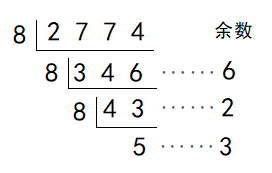安卓手机扫描二维码安装App

### 进位制

（1）用0、1、2、3、4、5、6、7、8、9这十个数字（数码）表示；

（2）逢十进一.

﻿$(1111)_2$﻿ ﻿$=$﻿ ﻿$1 \times 2^3$﻿ ﻿$+ 1 \times 2^2$﻿ ﻿$+ 1 \times 2^1$﻿ ﻿$+1$﻿ ﻿$=15$﻿

﻿$(\overline{a_0 a_1 \cdots a_n})_g$﻿ ﻿$=a_0 \times g^n$﻿ ﻿$+a_1 \times g^{n-1}$﻿ ﻿$+ \cdots$﻿ ﻿$+ a_{n-1} \times g$﻿ ﻿$+ a_n$﻿﻿$2774 =$﻿ ﻿$5 \times 8^3$﻿ ﻿$+3 \times 8^2$﻿ ﻿$+ 2 \times 8$﻿ ﻿$+6$﻿ ﻿$=(5326)_8$﻿苹果手机扫描二维码安装App